## OpenPIV tutorial of various correlation types¶

In this notebook we compare the time to run the same analysis using Cython (precompiled) version with the Python process using FFT and/or direct cross-correlation method

In :
from openpiv import tools, scaling, pyprocess, validation, filters
import numpy as np
import pylab
%matplotlib inline

In :
frame_a  = tools.imread( '../test1/exp1_001_a.bmp' )
frame_b  = tools.imread( '../test1/exp1_001_b.bmp' )

pylab.imshow(np.c_[frame_a,np.ones((frame_a.shape,20)),frame_b],
cmap=pylab.cm.gray)

Out:
<matplotlib.image.AxesImage at 0x7fdc2ac1f3d0>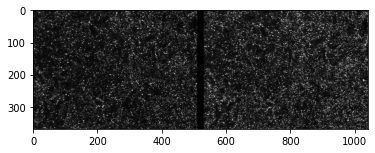In :
# Typical set of parameters

window_size = 32 # pixels, IW size in frame A
overlap = 16 # 50% overlap
search_area_size = 32 # pixels, IW in frame B, helps for large displacements
dt = 0.02 # second, 50 Hz, just an example
scaling_factor = 96.52 # micron/pixel

In :
%%time

# default correlation is FFT circular type (faster, less robust)
# default type of correlation is not normalized, faster
# we do not know the values of signal to noise ratio a priori
# therefore we decide that we remove lowest 5%
# the signal to noise ratio is defined here as 1st to 2nd peak ratio
# All the parameters need to be checked.

# get the values of displacements in pixel/sec units
u, v, sig2noise = pyprocess.extended_search_area_piv(
frame_a, frame_b,
window_size=window_size,
overlap=overlap,
dt=dt,
search_area_size=search_area_size,
sig2noise_method='peak2peak' )

# prepare centers of the IWs to know where locate the vectors
x, y = pyprocess.get_coordinates(frame_a.shape,
search_area_size=search_area_size,
overlap=overlap)

u, v, mask = validation.sig2noise_val( u, v,
sig2noise,
threshold = np.percentile(sig2noise,5))

# removing and filling in the outlier vectors
u, v = filters.replace_outliers( u, v, method='localmean',
max_iter=10,
kernel_size=2)

# rescale the results to millimeters and mm/sec
x, y, u, v = scaling.uniform(x, y, u, v,
scaling_factor=scaling_factor )

# save the data
x, y, u, v = tools.transform_coordinates(x, y, u, v)
tools.save(x, y, u, v, mask, 'circular_default.txt' )

CPU times: user 107 ms, sys: 12 ms, total: 119 ms
Wall time: 118 ms

/home/user/miniconda3/envs/openpiv/lib/python3.8/site-packages/numpy/core/fromnumeric.py:3419: RuntimeWarning: Mean of empty slice.
return _methods._mean(a, axis=axis, dtype=dtype,
/home/user/miniconda3/envs/openpiv/lib/python3.8/site-packages/numpy/core/_methods.py:188: RuntimeWarning: invalid value encountered in double_scalars
ret = ret.dtype.type(ret / rcount)

In :
%%time

# use normalized_correlation
# both image intensity is normalized before correlation
# and the correlation map has peaks between 0..1

# get the values of displacements in pixel/sec units
u, v, sig2noise = pyprocess.extended_search_area_piv(
frame_a, frame_b,
window_size=window_size,
overlap=overlap,
dt=dt,
search_area_size=search_area_size,
sig2noise_method='peak2peak',
normalized_correlation = True)

# prepare centers of the IWs to know where locate the vectors
x, y = pyprocess.get_coordinates(frame_a.shape,
search_area_size=search_area_size,
overlap=overlap)

u, v, mask = validation.sig2noise_val( u, v,
sig2noise,
threshold = np.percentile(sig2noise,5))

# removing and filling in the outlier vectors
u, v = filters.replace_outliers( u, v, method='localmean',
max_iter=10,
kernel_size=2)

# rescale the results to millimeters and mm/sec
x, y, u, v = scaling.uniform(x, y, u, v,
scaling_factor=scaling_factor )

# save the data
x, y, u, v = tools.transform_coordinates(x, y, u, v)
tools.save(x, y, u, v, mask, 'circular_normalized.txt' )

CPU times: user 157 ms, sys: 0 ns, total: 157 ms
Wall time: 156 ms

In :
%%time

# change to another type of correlation 'linear' - uses
# zero padding prior to the correlation
# it requires uniform background and therefore
# we need to normalize intensity of the images

u, v, sig2noise = pyprocess.extended_search_area_piv(
pyprocess.normalize_intensity(frame_a),
pyprocess.normalize_intensity(frame_b),
window_size=window_size,
overlap=overlap,
dt=dt,
search_area_size=search_area_size,
sig2noise_method='peak2peak',
correlation_method = 'linear')

# prepare centers of the IWs to know where locate the vectors
x, y = pyprocess.get_coordinates(frame_a.shape,
search_area_size=search_area_size,
overlap=overlap)

u, v, mask = validation.sig2noise_val( u, v,
sig2noise,
threshold = np.percentile(sig2noise,5))

# removing and filling in the outlier vectors
u, v = filters.replace_outliers( u, v, method='localmean',
max_iter=10,
kernel_size=2)

# rescale the results to millimeters and mm/sec
x, y, u, v = scaling.uniform(x, y, u, v,
scaling_factor=scaling_factor )

# save the data
x, y, u, v = tools.transform_coordinates(x, y, u, v)
tools.save(x, y, u, v, mask, 'linear_intensity.txt' )

CPU times: user 188 ms, sys: 13.1 ms, total: 201 ms
Wall time: 200 ms

In :
%%time

# add normalized correlation to linear

u, v, sig2noise = pyprocess.extended_search_area_piv(
pyprocess.normalize_intensity(frame_a),
pyprocess.normalize_intensity(frame_b),
window_size=window_size,
overlap=overlap,
dt=dt,
search_area_size=search_area_size,
sig2noise_method='peak2peak',
correlation_method='linear',
normalized_correlation=True)

# prepare centers of the IWs to know where locate the vectors
x, y = pyprocess.get_coordinates(frame_a.shape,
search_area_size=search_area_size,
overlap=overlap)

u, v, mask = validation.sig2noise_val( u, v,
sig2noise,
threshold = np.percentile(sig2noise,5))

# removing and filling in the outlier vectors
u, v = filters.replace_outliers( u, v, method='localmean',
max_iter=10,
kernel_size=2)

# rescale the results to millimeters and mm/sec
x, y, u, v = scaling.uniform(x, y, u, v,
scaling_factor=scaling_factor )

# save the data
x, y, u, v = tools.transform_coordinates(x, y, u, v)
tools.save(x, y, u, v, mask, 'linear_normalized.txt' )

CPU times: user 187 ms, sys: 11.1 ms, total: 198 ms
Wall time: 197 ms

In :
%%time

# change to the extended search_type when window size in B is
# larger to capture large displacements

search_area_size = 40

u, v, sig2noise = pyprocess.extended_search_area_piv(
frame_a,
frame_b,
window_size=window_size,
overlap=overlap,
dt=dt,
search_area_size=search_area_size,
sig2noise_method='peak2peak',
correlation_method='linear',
normalized_correlation=True)

# prepare centers of the IWs to know where locate the vectors
x, y = pyprocess.get_coordinates(frame_a.shape,
search_area_size=search_area_size,
overlap=overlap)

u, v, mask = validation.sig2noise_val( u, v,
sig2noise,
threshold = np.percentile(sig2noise,5))

# removing and filling in the outlier vectors
u, v = filters.replace_outliers( u, v, method='localmean',
max_iter=10,
kernel_size=2)

# rescale the results to millimeters and mm/sec
x, y, u, v = scaling.uniform(x, y, u, v,
scaling_factor=scaling_factor )

# save the data
x, y, u, v = tools.transform_coordinates(x, y, u, v)
tools.save(x, y, u, v, mask, 'linear_normalized_extended.txt' )

CPU times: user 189 ms, sys: 2.48 ms, total: 192 ms
Wall time: 191 ms

In :
%%time

# default correlation is FFT circular type (faster, less robust)
# default type of correlation is not normalized, faster
# we do not know the values of signal to noise ratio a priori
# therefore we decide that we remove lowest 5%
# the signal to noise ratio is defined here as 1st to 2nd peak ratio
# All the parameters need to be checked.

# get the values of displacements in pixel/sec units
u, v, sig2noise = pyprocess.extended_search_area_piv(
frame_a, frame_b,
window_size=window_size,
overlap=overlap,
dt=dt,
search_area_size=search_area_size,
sig2noise_method='peak2peak' )

# prepare centers of the IWs to know where locate the vectors
x, y = pyprocess.get_coordinates(frame_a.shape,
search_area_size=search_area_size,
overlap=overlap)

u, v, mask = validation.sig2noise_val( u, v,
sig2noise,
threshold = np.percentile(sig2noise,5))

# removing and filling in the outlier vectors
u, v = filters.replace_outliers( u, v, method='localmean',
max_iter=10,
kernel_size=2)

# rescale the results to millimeters and mm/sec
x, y, u, v = scaling.uniform(x, y, u, v,
scaling_factor=scaling_factor )

# save the data
x, y, u, v = tools.transform_coordinates(x, y, u, v)
tools.save(x, y, u, v, mask, 'circular_extended.txt' )

CPU times: user 81 ms, sys: 0 ns, total: 81 ms
Wall time: 80.9 ms

In :
tools.display_vector_field('linear_normalized_extended.txt', scale=30)
tools.display_vector_field('linear_normalized.txt', scale=30)
tools.display_vector_field('linear_intensity.txt', scale=30)
tools.display_vector_field('circular_default.txt', scale=30);
tools.display_vector_field('circular_normalized.txt', scale=30);
tools.display_vector_field('circular_extended.txt', scale=30);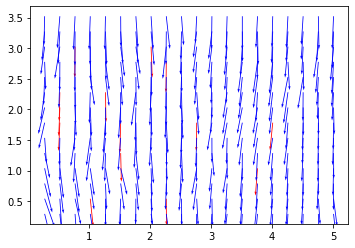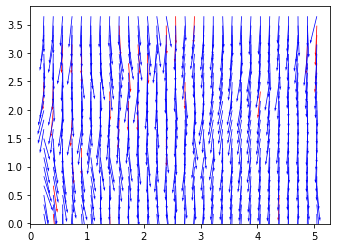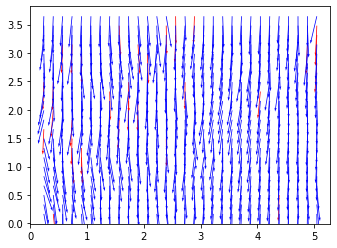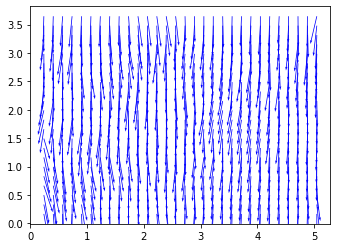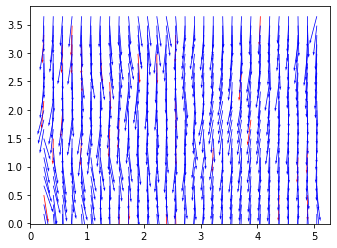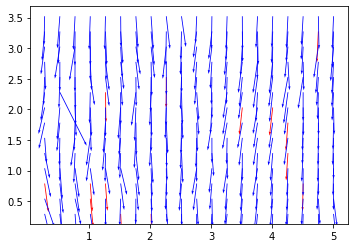In [ ]:


In [ ]:


In [ ]: